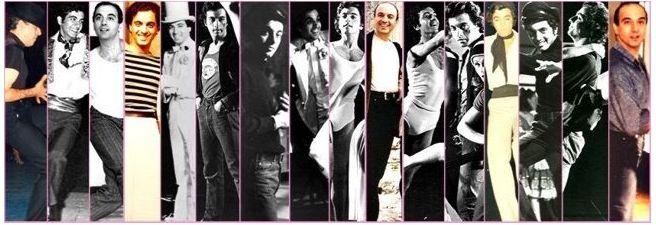*  I n t e r n a t i o n a l   a d j u d i c a t o r .

*  W o r l d   d a n c e   m a s t e r  i n   A m e r i c a n   s t y l e   B a l l r o o m   a n d   L a t i n .

*  A w a r d e d   c h o r e o g r a p h e r .

(())  * * * * * * * * * * * *  * * * * * * * * * *  (())

C e r t i f i e d   W o r l d   M a s t e r   D a n c e   I n s t r u c t o r   W i t h . . .

G E N E   K E L L Y   Australia. Bronze, Silver, Gold 1968 -1974.

F R E D   A S T A I R E   Bronze, Silver, Gold awards 1968 -1973.

A R T H U R   M U R R A Y   Bronze, Silver, Gold awards 1971-1973.

C E L E B R I T Y   S T U D I O S   International, Bronze, Silver, Gold awards 1978 -1980.

U.K.A   International Bronze, Silver 1994

A R T H U R   M U R R A Y   updated, Bronze, Silver, Gold, Gold Bar 1984-1990.

F R E D   A S T A I R E   updated, Bronze, Silver, Gold 1999 -2001.

I.D.T.A   Great Britain  Full Member in Ballroom 2006.

I.D.T.A   Great Britain   Full Member in latin 2006

A r t i s t i c   A c h i e v e m e n t s .

D O R A   S T R A T O U   Greek folk dances childrens group 1957-1963

A U S T R A L I A N   D A N C E   T H E A T E R   Modern and Jazz Ballet Soloist 1967 -1974.

A U S T R A L I A N   T E L E V I S I O N   Presenter, Dancer, Choreographer 1967 -1985.

A R T H U R   M U R R A Y   Dance director, Sydney Australia 1970 -1973.

G R E E K   M O V I E S,  T H E A T E R,  T E L E V I S I O N   Dancer, choreographer 1974 -1980.

T H E   F I R S T   G R E E K   A D J U D I C A T O R   To judge a World showdance competition 2003

S p e c i a l    A w a r d s .

A R T H U R   M U R R A Y   Ten dance Australian Champion 1971 -1973.

A R T H U R  M U R R A Y   Dance-o-rama winner 1970 & 1971

A U S T R A L I A N   S T A T E   Choreography award 1971.

F R E D   A S T A I R E   Tap dance award 1975.

F R E D   A S T A I R E   Exhibition award 1999.

N A T I O N A L   D A N C E   C O U N C I L   O F   G R E E C E   Special Award for his contribution to dance, in Greece 1999

U.S.A   Choreography award 2000..

U.S.A   New York.Tap dance award 2004

C r e a t i o n s .

B A L L R O O M   D A N C E   C O M P A N Y   Australia 1976 -1985.

F R E D'S   A M E R I C A N   D A N C E   C E N T E R   First  Dance Studio 1987.

D A N C I N'   I N T E R N A T I O N A L   Dance Studio 1998.

E A S Y D A N C E   Dance studio 2002.

F R E D S   A M E R I C A N   D A N C E   C E N T E R   New  Dance Studio and "Head office" in Pagrati Athens  2006

C o - C r e a t i o n s .

T H E   F I R S T   E V E R  "  A M E R I C A N   S T Y L E  "   B A L L R O O M   A N D   L A T I N   D A N C E   S T U D I O   I N   G R E E C E.

The people who made it possible are,  F r a n k   K e l l y,  D e s m a   K e l l y a n d   G e o r g e   S a y a s  w a y   b a c k   i n   1 9 7 4.

(())  * * * * * * * * * * * * * * * * * * * * * * * * * * * * * *  (())

[Home] [Dances we teach] [Teaching Methods] [Our Programs] [F.A.Q.] [Benefits of Dance] [Wedding Dance] [Freds Children] [Directors Bio] [Contact us]

* *